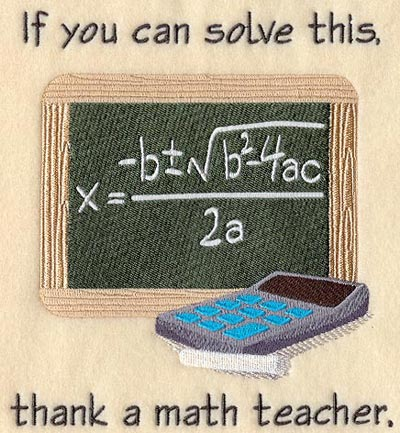Learn the 4 Things You Must Know Before Taking the GMAT!   Download it Now!A lot of GMAT students strongly dislike algebra. Hate is a strong word, but you get my drift. Moreover, of all the algebra topics tested on the GMAT, quadratic equations tend to give students the most pause. You may be one of those students. Well, fear not! I’m about to make your life a whole lot easier — at least as it pertains to data sufficiency questions on the GMAT quantitative section.

Remember that quadratic equations are polynomial equations of the second degree, meaning that they contain a squared term. They follow this general form:

ax² + bx + c = 0

where x represents a variable, or unknown, and a, b, and c are constants.

On GMAT problem solving questions, you may be asked to find the “roots” of a quadratic equation, i.e. the possible values of x. Sometimes that’s pretty straightforward, such as in the following equation:

x² = 36

Without much calculation, you intuitively know that x could equal 6 or -6.

A more difficult quadratic equation could look something like this:

2x² + 9x – 5 = 0

To find the possible values of x in that equation, you’d have to factor it. I recommend the “reverse FOIL” method that we teach in our video lesson “Quadratic Equations, Simultaneous Equations, and Inequalities“. (Nice job if you figured out that x=1/2 or x=5).

The best part about data sufficiencies on the GMAT is that you don’t actually have to solve anything! Remember, all you’re trying to do is determine whether or not you could solve the question, given the information in the statement(s). I always tell my students to ask themselves when they’re looking at a statement: Could a Harvard math professor with a calculator and enough time solve the question, given the information provided?

When it comes to quadratic equations, that should be a very reassuring thought! If you had trouble factoring either of the sample quadratic equations above, for example, that’s a non-issue when it comes to data sufficiencies. Most of the time you won’t actually have to find the possible values of x; rather, you simply need to determine whether or not you could.

Take a look at this sample data sufficiency question from a real former GMAT:

What is the value of x?
(1) 4x2 – 4x = -1
(2) 2x2 + 9x = 5

First things first, you should always put quadratic equations in their more recognizable form, set equal to 0. So, re-written, the question would look like this:

What is the value of x?
(1) 4x2 – 4x + 1 = 0
(2) 2x2 + 9x -5 = 0

Now, how do you know if the statements give you enough information to definitively solve for x, either individually or together? Remember that for a statement to be sufficient in this example, it must yield EXACTLY one possibly value for x. In my example above where x2=36, for example, that would NOT be sufficient to solve definitively for x because that equation yields TWO possible values for x, 6 and -6.

So, you could certainly reverse FOIL the equations in each statement, find the roots, and determine if there’s only 1 possible value of x in either equation.

OR… you can use this shortcut:

Remember the generic form of a quadratic equation:

ax² + bx + c = 0

Here’s the shortcut:

1) If b2 – 4ac > 0, there are two (2) real roots (i.e. two possible values of x)
2) If b2 – 4ac = 0, then there is exactly one (1) real root (also called a double root)
3) If b2 – 4ac < 0, then there are no real roots

So, let’s re-visit our example from above, looking at each statement in turn:

(1) 4x2 – 4x + 1 = 0

Applying the shortcut, (-4)2 – 4(4)(1) = 16 – 16 = 0

So, in that quadratic equation, there is only one (1) value for x. Therefore the statement IS sufficient to answer the question. Do we care what x equals? No! We simply care that we could definitely determine it, as this shortcut reveals.

No let’s look at statement (2):

(2) 2x2 + 9x – 5 = 0

Applying the shortcut, 92 – 4(2)(-5) = 81 + 40 = 121. Since 121 is greater than zero, there are two (2) possible values of x, so that statement is NOT sufficient to definitively solve for x.

Therefore, the answer to this data sufficiency problem is A. Great job if you got that!

Hopefully you see how this shortcut can save you time and stress. Learn it, practice it, and go out and dominate the GMAT!